Electron. J. Differential Equations, Vol. 2019 (2019), No. 90, pp. 1-32.

### Existence of solutions for non-local elliptic systems with Hardy-Littlewood-Sobolev critical nonlinearities Yang Yang, Qian Yu Hong, Xudong Shang

Abstract:
In this work, we establish the existence of solutions for the nonlinear nonlocal system of equations involving the fractional Laplacian,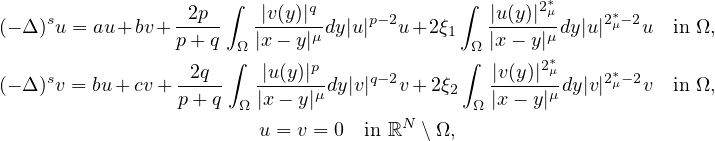where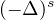is the fractional Laplacian operator,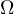is a smooth bounded domain in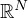,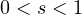,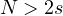,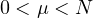,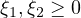,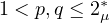and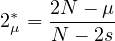is the upper critical exponent in the Hardy-Littlewood-Sobolev inequality. The nonlinearities can interact with the spectrum of the fractional Laplacian. More specifically, the interval defined by the two eigenvalues of the real matrix from the linear part contains an eigenvalue of the spectrum of the fractional Laplacian. In this case, resonance phenomena can occur.

Submitted December 11, 2018. Published July 19, 2019.
Math Subject Classifications: 35R11, 35R09, 35A15.
Key Words: Fractional Laplacian; Choquard equation; Linking theorem; Hardy-Littlewood-Sobolev critical exponent; Mountain Pass theorem.

Show me the PDF file (482 KB), TEX file for this article.Yang Yang School of Science Jiangnan University Wuxi, Jiangsu 214122, China email: yynjnu@126.com Qian Yu Hong School of Science Jiangnan University Wuxi, Jiangsu 214122, China email: 1031369190@qq.com Xudong Shang School of Mathematics Nanjing Normal University, Taizhou College Taizgou, Jiangsu 225300, China email: xudong-shang@163.com